Download Presentation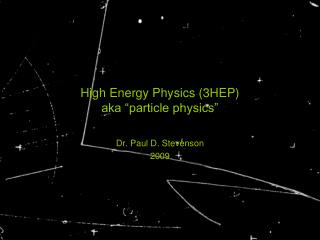High Energy Physics (3HEP) aka “particle physics”

# High Energy Physics (3HEP) aka “particle physics” - PowerPoint PPT Presentation

High Energy Physics (3HEP) aka “particle physics”. Dr. Paul D. Stevenson 2009. Outline of Course. Relativistic kinematics. Particle detectors, beam characteristics.I am the owner, or an agent authorized to act on behalf of the owner, of the copyrighted work described.
Download Presentation## High Energy Physics (3HEP) aka “particle physics”

An Image/Link below is provided (as is) to download presentation

Download Policy: Content on the Website is provided to you AS IS for your information and personal use and may not be sold / licensed / shared on other websites without getting consent from its author.While downloading, if for some reason you are not able to download a presentation, the publisher may have deleted the file from their server.

- - - - - - - - - - - - - - - - - - - - - - - - - - E N D - - - - - - - - - - - - - - - - - - - - - - - - - -
Presentation Transcript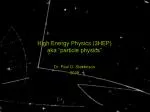### High Energy Physics (3HEP)aka “particle physics”

Dr. Paul D. Stevenson

2009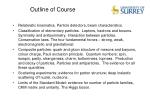Outline of Course
• Relativistic kinematics. Particle detectors, beam characteristics.
• Classification of elementary particles. Leptons, hadrons and bosons. Symmetry and anitsymmetry. Interaction between particles. Conservation laws. The four fundamental forces – strong, weak, electromagnetic and gravitational;
• Composite particles: quark and gluon structure of mesons and baryons, colour charge, Pauli exclusion principle. Quantum numbers: spin, isospin, parity, strangeness, charm, bottomness, topness. Production and decay of particles. Particles and antiparticles. The evidence for all these quantities.
• Scattering experiments; evidence for parton structure; deep inelastic scattering off nuclei, nucleons;
• Limits of the Standard Model: evidence for number of particle families, CKM matrix and unitarity. The Higgs boson.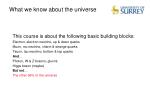What we know about the universe

This course is about the following basic building blocks:

Electron, electron neutrino, up & down quarks

Muon, mu neutrino, charm & strange quarks

Tauon, tau neutrino, bottom & top quarks

And…

Photon, W & Z bosons, gluons

Higgs boson (maybe)

But not…

The other 96% of the universe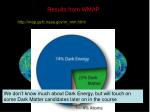Results from WMAP

http://map.gsfc.nasa.gov/m_mm.html

We don’t know much about Dark Energy, but will touch on some Dark Matter candidates later on in the course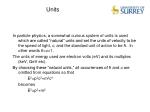Units

In particle physics, a somewhat curious system of units is used which are called “natural” units and set the units of velocity to be the speed of light, c, and the standard unit of action to be ħ. In other words ħ=c=1.

The units of energy used are electron volts (eV) and its multiples (keV, GeV etc).

By choosing these “natural units,” all occurrences of ħ and c are omitted from equations so that

E2=p2c2+m2c4

becomes

E2=p2+m2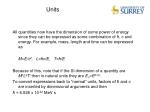Units

All quantities now have the dimension of some power of energy since they can be expressed as some combination of ħ, c and energy. For example, mass, length and time can be expressed as

M=E/c2, L=ħc/E, T=ħ/E

Because of this, note that if the SI dimension of a quantity are MpLqTr then in natural units they are En=Ep-q-r.

To convert expressions back to “normal” units, factors of ħ and c are inserted by dimensional arguments and then

ħ = 6.528 x 10-22 MeV s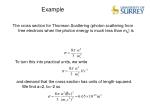Example

The cross section for Thomson Scattering (photon scattering from free electrons when the photon energy is much less than me) is

To turn this into practical units, we write

and demand that the cross section has units of length-squared. We find a=2, b=-2 soCollisions and Decays

Most experimental particle physics is concerned with collisions and decays.

Collisions: a+b->c+d

Given the initial particles we can have different final states: these are called channels.

We can observe many things in a given collision: the directions of c and d, their polarisation etc. We are interested also in the cross-section of the process. For each different channel we can define a partial cross-section.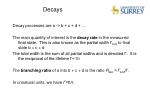Decays

Decay processes are a -> b + c + d + …

The main quantity of interest is the decay rate in the measured final state. This is also known as the partial width Γbcd to final state b + c + d

The total width is the sum of all partial widths and is denoted Γ. It is the reciprocal of the lifetime Γ=1/τ

The branching ratio of a into b + c + d is the ratio Rabc = Γbcd/Γ.

In unnatural units, we have Γ=ħ/τ.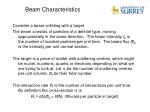Beam Characteristics

Consider a beam colliding with a target

The beam consists of particles of a definite type, moving approximately in the same direction. The beam intensity Ib is the number of incident particles per unit time. The beam flux Φb is the intensity per unit normal section.

The target is a piece of matter with scattering centres, which might be nuclei, nucleons, quarks or electrons depending on what we are trying to look at. Let nt be the number of scattering centres per unit volume, Nt be their total number

The interaction rate Ri is the number of interactions per unit time. By definition the cross-section σiis

Ri = σNtΦb = WNt. (W=rate per particle in target)Antiparticles

Assume that a particle in free space is described by a de Broglie wavefunction:

Then using E=p2/2m, it is seen that the wavefunction obeys the Schrödinger equation:

But we now know (from relativity) that E2=p2c2+m2c4

So what is a suitable form for a relativistic wave equation?Klein-Gordon Equation

But for any plane wave solution, there is an equivalent one with the opposite sign of energy.

The negative energy solutions are a consequence of the quadratic mass-energy relations and cannot be avoided

Dirac extended the above equation to apply to spin-1/2 objects (e.g. electrons), and the results agreed impressively with experiment (e.g by predicting the correct magnetic moment).Dirac Hole Theory

The negative energy states remained, and Dirac picture the vacuum as a “sea” of negative energy electron states combining to give a total energy, momentum and spin of zero.

Vacuumstatemeans negative energy states filled with electronsPositrons

When a “hole” is created in the negative energy sea, Dirac postulated that this corresponds to a particle with positive charge, but with mass equal to the electron, called the positron or anti-electron.

γNote that removing an electron with energy E=-Ep<0, momentum -p, and charge -e from the vacuum (which has E=0, p=0, Q=0) leaves the state with a positive energy and momentum and with a positive charge.
• This state cannot be distinguished in any measurement from the situation in which an equivalent positive energy particle is added to the system.
• Dirac postulated the existence of the positron based on this hole theory in 1928
• Not everyone took this idea seriously:
• “Dirac has tried to identify holes with antielectrons … We do not believe that this explanation can be seriously considered” (Handbuch der Physik, 24, 246 (1933))
• The positron was discovered experimentally in 1933Antiparticles in General
• Any particle with spin-1/2 obeys the Dirac equation, and the hole theory applies. This means every spin-1/2 particle has an antiparticle.
• Spin-0 particles do not obey the Dirac equation and do not feel the Pauli Exclusion Princliple on which hole-theory depends, and do not (necessarily) have antiparticles.

Need to extend the list of fundamental particles:Leptons

The fundamental fermions (particles with spin-1/2) which do not feel the strong force are called leptons. They are

§ neutrino masses non-zero but small. See later. # neutrinos do not decay, but oscillate. see later, too.Interactions

The charged leptons interact via the electromagnetic interaction (like all charged particles) and the weak interaction (like all particles)

The uncharged leptons (i.e. the neutrinos) interact only via the weak interaction, mediated by W and Z bosons

In all interactions, it is observed that the following numbers are conserved:

Le = N(e-) - N(e+) + N(ve) - N( )

Lµ = N(µ-) - N(µ+) + N(vµ)-N( )

L = N(-) - N(+) + N(v) - N( )Lepton processes

Since neutrinos do not feel the electromagnetic interaction, in electromagnetic processes, the Le conservation rule reduces to the conservation of N(e-)-N(e+). This implies that in electromagnetic processes, electrons and positrons can only be created or annihilated in pairs.

In weak interactions, other possibilities exist. For example, the following beta-decay process is allowed:

n → p + e- +

because the total Le number on the left (0) is equal to the number on the right (1-1=0)

Charge is also conserved, which is required in all physical processesDisallowed processes

Various processes are allowed by energy conservation, but not by some of the empirical conservations laws, e.g.Neutrino interactions

Neutrinos are very hard to detect, because they interact only via the weak interaction. This means that the scattering amplitude (determining the probability of reactions to occur) is small because of the large mass of the W and Z particles. Its existence was postulated in 1930 by Fermi to appear in ß-decay.

If ß-decay had a two-body final state (Z,N) → (Z+1,N-1) + e-then the energy of the electron would be uniquely determined by

However, experimentally, electrons are observed to have a range of energies. If a neutrino is also present the energy of the electron can lie in the rangeNeutrino detection

Though postulated in 1930, neutrinos were not detected until 1956. They can be detected in the following processes:

These have a cross section of around 10-47m2 which means that a neutrino would typically have to travel through many light years of matter before interaction. If the neutrino flux is large enough, however, sufficient events can be seen in much smaller (!) detectors.

see article from G. L. Trigg, Landmark Experiments in 20th Century PhysicsHeavier Leptons

electrons are the lightest charged particles, and as such can not decay (since charge must be conserved).

Muons behave very much like electrons, except that their mass is much larger (105.7 MeV/c2 compared to 0.511 MeV/c2).

In particular, they satisfy the Dirac equation for point-like spin-1/2 particles, and their magnetic moment is

µ=(e/mµ)S

indicating that there is no substructure, and the muon is an elementary particle.Lepton Decay

Muons decay with a lifetime of 2.2x10-6s via

Taus decay to many different final states. This is because there mass is sufficiently high that many different allowed combinations of particles have a lower rest-mass energy than the tau.

The different decays are characterised by their branching ratio, which gives the fraction of decays to a given final state compared to all decays. For taus we haveLepton Universality

electrons are light and stable, and are stopped by a modest thickness of lead. muons are about 200 times heavier and are very penetrating. taus are much heavier still and has a lifetime many orders of magnitude below the muon. Nevertheless, all experimental data is consistent with the assumption that the interactions of the electron with its neutrino, the muon with its neutrino and the tau with its neutrino are identical, provided the mass difference is taken into account. This is know as Lepton Universality.

e.g. the decay rate for a weak process is predicted to be proportional to the Q-value multiplied by the same GF2 independent of kind of lepton: for example,

In excellent agreement with experimentNeutrino Mass

The masses of particles is not predicted by the standard model. Neutrinos certainly have a very small mass (e.g. as measured by the electron energy distribution in beta decay, and had usually been considered to be zero. If the neutrino masses are not zero, neutrino mixing can occur.

This happens because the weak neutrino states (e) need not be the same as the mass neutrino states (123) but linear combinations of them. For simplicity, let’s consider the mixing of two states in the following way:Oscillations

Consider an electron neutrino created at time t=0 with momentum p as

After a time t it will be in a state

corresponding to a probability of having changed weak eigenstate ofDetection

When cosmic ray protons collide with atoms in the atmosphere, they create many pions which decay to neutrinos via

One would expect then to have twice as many muon neutrinos as electron neutrinos, but the observed ratio was about 1.3:1

Neutrinos do oscillate.Quarks

The six quark types, or flavours, know to exist are given below:Quarks

Unlike the leptons, quarks are never seen as free particles but only in the following combinations:

baryons: qqq

antibaryons:

mesons:

Collectively, these resulting particles are known as hadrons.

No other combination are seen, though it is thought that other possibilities could exist (e.g. pentaquark states)Quarks and Interactions

Quarks (and hence hadrons) feel the weak interaction (because all known particles do), the electromagnetic interaction (because they are charged) and also the strong interaction, mediated by gluons.

Despite the fact that they are never seen in isolation, the evidence for their existence is compelling because of:

• deep-inelastic scattering
• JetsSpectroscopy

Combining quarks in the allowed forms of baryons or mesons correctly reproduces all known hadrons, e.g.

p = uud

n = udd

• = uds

+ =

K+ =

B- =

etc.

Additive properties like charge are seen to agree straightforwardly with the quark model. A large part of the mass is associated with the binding energy, and is not easy to calculate.

Young man, if I could remember the names of all these particles, I would have been a botanist -- Enrico FermiVarious quantum numbers are associated with hadrons. Some are related to symmetry operations, and some are intrinsic to the quarks. The quark quantum numbers are the electric charge Q, and baryon number B, which are conserved in all known interactions, and the strangeness S, charm C, beauty B’ and truth T, which are conserved in strong and electromagnetic, but not in weak, interactionsIsospin

For partly historical reasons, the internal quantum number associated with the up and down quarks works a little differently.

Heisenberg thought that the similarity in mass between the neutron and the proton meant that there was some underlying symmetry which was only broken by the charge on the proton vs the lack of charge on the neutron. He called this isospin symmetry, because it behaves like an angular momentum, and thought of the nucleon as an isospin-1/2 system, with the proton being the +1/2 projection and the neutron the -1/2 projection on the third axis.

Similarly for other families of particles with similar masses and other properties:quark isospin

u & d quarks are an isodoublet, both with I=1/2, but d has I3=Iz=-1/2, u has I3=Iz=+1/2

the equivalent antiquarks are also an isodoublet with I=1/2, but with opposite third-components.

Another quantum number is often used, which is derived from the known ones, called hypercharge:

Y=B+S+C+B’+T

and then for a hadron, I3=Q-Y/2Example: How new particles can be identified by the quantum numbers and conservation laws.

When a K- is fired at a proton target, they can interact (with a cross section appropriate to the strong interaction to give an - and a new particle +, which decays with weak interaction lifetimes to + + + n, or + 0 + p.

Applying conservation of B,S,C,B’,T to the K-+p  - + + reaction, and using the known values for K- (B=0, S=-1, C=B’=T=0), p ( B=1, S=0, C=B’=T=0) and - (B=0, S=C=B’=T=0) tells us that for the +, B=1, S=-1, C=B’=T=0. From the relationship between hypercharge and isospin, we also infer I3=1. It must therefore be part of an isotriplet, and partners of similar mass but different isospin should exist, as indeed they do.

We can infer the quark structure from the quantum numbers as uus (n.b components of vectors are always additive)Space-Time Symmetries

As well as the quantum numbers associated directly with intrinsic quark properties, particles (including the leptons) have quantum numbers associated with spatial properties. These are Spin, J, Parity, P, and Charge Conjugation, C.

SPIN

We use the word spin to characterise the intrinsic angular momentum of a particle whether or not it is composite.

If the particle is elementary, the total spin J is equal to the intrinsic spin S, e.g. 1/2 for any of the fundamental fermions (quarks & leptons)Meson Angular Momentum

Mesons are made a quark and an antiquark which have intrinsic spin of their own, and can have orbital angular momentum about their centre of mass

If we first consider the case that there is no orbital angular momentum (this is the case for the lowest masss mesons) then we just have J=S and S is made by coupling together two single spins of 1/21.

When coupling an angular momentum I1 to another I2 the allowed values of the result I areI = |I1-I2|, |I1-I2|+1,…,I1+I2-1, I1+I2

Coupling 1/2 with 1/2 gives only 0 or 1 as possibilities for S and hence for J when L=0.

1 we are not including units of c or hbar. Angular momentum has units of hbarMeson Angular Momentum

If the quark and antiquark in the meson have a relative angular momentum (which is quantized in integer units of hbar) L=1, 2, 3, … then this L couples with the coupled intrinsic spin S according to the normal rules of AM coupling, I.e. the total spin J can lie anywhere (in integer steps) between |L-S| and L+S.

We label the mesons in spectroscopic notation:

2S+1LJ

where L is written as the usual identifying letter: S=0, P=1, D=2, F=3, G=4 etc.. and S and J are given as numbers. So a pion, which has L=S=0 has an angular momentum configuration 1S0 . For a given quark-antiquark pair, there are many allowed angular momentum configurations, which are identified as different particles. For example there is a state made of u (like a +) but with J=1, which is labelled as +. It can be thought of as an excited state of +, but since it has different quantum numbers, it is also thought of as a particle in its own right.Baryon Spin

In the case of baryons, the three spins of 1/2 can couple to either 3/2 or 1/2 which then couples to the orbital angular momentum, which can be any integer value.

q3

q1

L3

L=L12+L3

L12

q2

what are the allowed baryon states for L=0, L=1?Parity

The parity transformation is xixi’=-xi, i.e. the position vector of every particle is reflected in the origin. If we define a parity operator P as

P(x,t) = Pa(-x,t)

where Pa is a phase factor. Since acting with the parity operator twice must give us back the original state, we have

P2(x,t) = (x,t) =Pa2(x,t)

and so Pa=1Intrinsic Parity

Consider an eigenfunction of momentum

then

so when a particle is at rest (p=0), it is an exact eigenstate of the parity operator with eigenvalue Pa. For this reason, Pa is called the intrinsic parity of particle a, or more commonly just the parity of particle a.

If a Hamiltonian is invariant under the parity transformation then [H,P]=0 and in a reaction governed by the Hamiltonian, the initial and final parities must be the same, and parity is conserved. This is true in the strong and electromagnetic interactions, but not the weak interaction.Angular Momentum & Parity

orbital angular momentum wavefunction are written in spatial coordinates as spherical harmonics in the angular part. These feature in the usual separable solutions to central-field potential in quantum mechanics:

(r,,) = R(r)Ylm(,)

The first few spherical harmonics are

Y00 = 1/sqrt(4)

Y10 = sqrt(3/4) cos

Y11 = -sqrt(3/8)sin exp(i)Spherical Harmonics

Using x=rsincos, y=rsinsin, z=rcos

the parity transformation in polar coordinates become

r  r’ = r

•  ’ = -
•  ’ = +

from which it can be shown that

Ylm(,)  Ylm(-, +) =(-1)l Ylm(,)

and (r,,) is an eigenstate of parity with eigenvalue Pa(-1)l (where Pa is the intrinsic parity ignoring angular momentum)Fermion Parity

From Dirac’s equation one can obtain the following for any fundamental fermion and its antiparticle

Parity is not conserved in the weak interaction. In the strong interaction quarks and antiquarks are always produced in pairs. Likewise the leptons and their anitleptons. One can indeed confirm the above relation experimentally but not determine the parity of the individual fermions. By convention the particles (quarks, electron etc.) are assigned P=+1 and the antiparticles (antiquarks, positron etc.) are then P=-1.

The parity of the photon can be derived from electromagnetic theory. It is P=-1Charge Conjugation

Charge conjugation is an operation which replaces all particles by their antiparticles in the same state (so that positions, momenta etc are unchanged).

Like Parity, Charge Conjugation is a good symmetry of the strong and electromagnetic interaction, but not the weak interaction. The Charge Conjugation quantum number of a particle is conserved in these interactions.

It can take on the values ±1. Its conservation can be used to understand why some reaction will work but not others, and help identify particles.0 decay

for a quark antiquark pair with given values of S, J, and L, the quark model predicts

It is observed that the dominant decay mode of the 0 is 0 . Then

So the final state has C=1, so too must the initial state, in agreement with the quark model prediction (since a 0, in the lightest multiplet of mesons, has L=S=0).

From electromagnetism, it can be deduced that C=-1. One does not see 0 

because of C-conservation in the electromagnetic interaction. Indeed, searches for this decay mode are made to look for potential C-parity breaking.Colo(u)r

The quark model is successful, but why are there no states of the form qq? Why is it always 3 quarks, 3 antiquarks or a quark-antiquark pair?

Also: according to quantum mechanics, the wavefunctions for composite particles made of identical spin-1/2 quarks must be antisymmetric under interchange of any two of the quarks. But the observed properties of some particles clearly contradict this.

e.g. the Ω- is observed to have strangeness S=-3 and spin J=3/2, L=0, indicating that it is made up of three identical strange quarks with their spins all pointing in the same direction, and in the same physical state. This wavefunciton is symmetric, and violates the Pauli principle.Colo(u)r

Another degree of freedom is at work. It is a property that quarks have but leptons do not, called color. It is in some sense analogous to electric charge, in that it has an associated color charge which is what the strong interaction acts on, like the electromagnetic interaction acts on charged things.

There are three kinds of color state for quarks, called red, green, and blue (which do not have anything to do with real colours). Antiquarks can be antired, antigreen or antiblue.

This solves the antisymmetry problem. In the Ω- the three quarks, labelled 1, 2 and 3 exist in a linear combination of different colour combinations which is antisymmetric with respect to the interchange of any two labels.Color confinement

It was hypothesised that hadrons could only exist in colorless states (either color-anticolor or rgb), which “explained” why only (anti-)baryons and mesons are seen.

Quarks are colored and have non-zero color charge and are hence confined to hadrons.

There is direct experimental evidence for color charge, coming from electron-positron annihilation (M&S 7.2)

Consider reactions of the type e+ + e- hadrons at rather high energies (15-40 GeV) in a colliding beam experimentJets

The primary mechanism of the reaction is that the electron and positron annihilate to a photon which then creates a (colourless) quark-antiquark pair.

Because the quarks are generated with so much momentum, and they are created moving away from each other, they soon fragment into two jets of hadrons. They cannot keep travelling as isolated quarks because of color confinement.

The total summed momentum for each jet lets one reconstruct the trajectory of each quark. This can be compared with the branch of

e+ + e- + + -Jet Distributions

From QED the differential cross-section for this process is well-known

to generate a quark-antiquark pair in this way (via the electromagnetic interaction), the cross section is just reduced slightly by the lower charges on the quarks compared to muons, but multiplied by a factor NC which is the number of different colours available for a quark of a given flavour. So the differential cross section for generation of a quark anti-quark of flavour a will becolour counting

If you perform this experiment, and measure these cross sections as a function of energy (and integrate over angle) you should see the ratio

Increase as more quark channels open up. For example, when u, d, s, c, b quarks are available, the ratio should be 11NC/9The Strong Interaction & QCD

QCD stands for Quantum ChromoDynamics and is the theory which governs the strong interaction.

The strong interaction acts by the exchange of gluons, which interact with objects with color charge, so in particular with quarks. Gluons are spin-1 bosons, which also have color charge, so gluons interact with themselves. This gives rise to curiosities such as glueball states which are made entirely of gluons. no such state has been conclusively observed, though there are some candidates.

In some circumstances the rather complicated QCD interactions can be approximated by a static potential between quarks (esp. if the quarks are heavy)quark potential

At short distances, the interaction between a heavy quark and its antiquark is dominated by single gluon exchange. This gives rise to a coulomb-like potential

V(r)=-(4/3) (S/r) for r<0.1 fm

because of color confinement, the strength of the interaction actually increases quite rapidly as you increase the distance between the quark and antiquark. This means a lot of energy is stored in the field which can turn into quark-antiquark pairs to stop the colored quarks from getting too far apart. Emprically the potential looks like:

V(r)rasymptotic freedom

The strong coupling constant S is not actually constant, but varies as a function of momentum transfer

At high values of momentum transfer (short distances) the coupling constant gets weaker. This means that when quarks are close to each other, the become more “free” from the strong force, but feel it very strongly when they try to separate.Strong interaction summary

The strong interaction acts between object with color. These are the quarks and gluons. The force is mediated by the exchange of gluons.

Conserved quantities in the strong interaction: Baryon number (B), quark flavour numbers: (S,C,T,B), parity (P), charge conjugation (C)

Also conserved (because all interactions conserve them): charge, angular momentum, energy.

Colored objects are never observed in isolation, but only combined into colorless particlesWeak Interaction with Hadrons
• There are two basic weak interaction vertices for interactions between W± and leptons:
• Which give rise to 8 basic reactions when pulled around in all possible time-orderingsLepton-Quark Symmetry

The basis of weak interactions with hadrons depends on how the W± bosons are absorbed or emitted by quarks.

For simplicity, we restrict discussion to the first two families of quarks: and

The two main ideas in the weak interaction of quarks are lepton-quark symmetry and quark mixing. They describe to a good accuracy the observed amplitudes of weak interaction events with W± bosons, and can deduce the interaction of quarks with the Z0 (see section 9.1.1 in Martin & Shaw for that).Lepton-Quark Symmetry

Lepton-Quark symmetry asserts that the first two generations of quarks, as on the previous slide, and the first two generations of leptons: & have identical weak interactions.

i.e. one obtains the basic vertices for weak interaction with quarks by the replacements:

leaving the coupling constants unchangedNeutron decay

and gud = gw = gcs

This idea works well in describing events such as neutron decay, and pion decay:

However it does not allow observed decays such as

(why?)

gw

W-

gud

d

d

u

u

d

uThe Cabibbo Hypothesis

To explain events such as K- decay, the idea of quark mixing was introduced. According to this idea, the d and s quarks take part in the weak interaction via the linear combinations

d’ = d cosC + s sinC

And

s’ = -d sinC + s cosC

where C is the Cabibbo mixing angle.

This means that lepton-quark symmetry is assumed to apply to the doublets andCabibbo angle

Now the previously allowed ‘udW’ vertex is suppressed by a factor cosC while the previously forbidden usW vertex is now allowed with a coupling gWsinC and the couplings are now:

gud = gcs = gwcosC

gus = -gcd = gwsinC

This set of couplings successfully accounts for the interaction of quarks with the W± - the so-called charged-current interactions (since the W± is the only charged force-carrying particle).

The success is demonstrated partly by the fact that the only free parameter - the Cabibbo angle - in this description can be measured in different ways, and the values agree with each otherMeasuring the Cabibbo Angle

From the relative decay rates of to one can determine the Cabibbo angle:

Of course, one must also take into account the difference in the d and s quark masses since this will have an effect on the rates. When this is done, the value is

giving C=12.7±0.1 degreesMeasuring the Cabibbo Angle

Comparing neutron and muon decay depends on the ratio

g2ud/g2w = cosC, which gives a value in agreement with that from K- / pi- decay.

Values of the Cabibbo angle should agree when obtained from the gcs coupling and the most accurate value for C coming from gcs is C = 12±1 degrees, which is compatible with the value from measuring gud.

Because of the value of the mixing angle, the couplings which were not allowed in the simple unmixed scheme are still heavily suppressed compared to the the allowed couplings. The decay rates for cabibbo-suppressed decays, which depend on the square of the couplings are suppressed by tan2 C≈ 1/20 compared to cabibbo-allowed decays. An example of this is that decays of particles with charmed quarks almost always have an s quark in the final state.Third Generation

The idea of quark mixing can be extended to the third generation with a mixing matrix

and apply lepton-quark symmetry to the doublets

, &

To a reasonable approximation Vtb=1 and the other elements in the third row and column are approximately zero